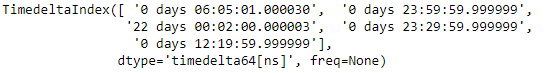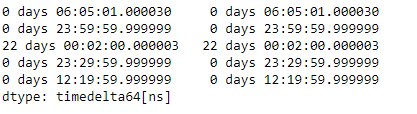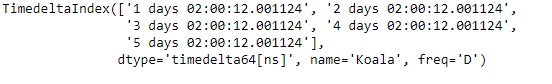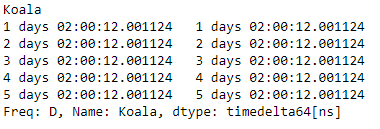Related Articles
Python | Pandas TimedeltaIndex.to_series()
• Last Updated : 06 Jan, 2019

Python is a great language for doing data analysis, primarily because of the fantastic ecosystem of data-centric python packages. Pandas is one of those packages and makes importing and analyzing data much easier.

Pandas` TimedeltaIndex.to_series()` function create a Series with both index and values equal to the index keys useful with map for returning an indexer based on an index.

Syntax : TimedeltaIndex.to_series(index = None, name = None)

Parameters :
index :index of resulting Series. If None, defaults to original index (Index, optional)
name :name of resulting Series. If None, defaults to name of original index (string, optional)

Return : Series : dtype will be based on the type of the Index values.

Example #1: Use `TimedeltaIndex.to_series()` function to construct a Series object from the given TimedeltaIndex object.

 `# importing pandas as pd``import` `pandas as pd`` ` `# Create the TimedeltaIndex object``tidx ``=` `pd.TimedeltaIndex(data ``=``[``'06:05:01.000030'``, ``'+23:59:59.999999'``,``                        ``'22 day 2 min 3us 10ns'``, ``'+23:29:59.999999'``,``                        ``'+12:19:59.999999'``])`` ` `# Print the TimedeltaIndex object``print``(tidx)`

Output :Now we will use the `TimedeltaIndex.to_series()` function to construct a Series object using tidx.

 `# return a series``tidx.to_series()`

Output :As we can see in the output, the `TimedeltaIndex.to_series()` function has returned a newly constructed Series object using the tidx object. Notice the index of the Series is also constructed using the labels of the given object.

Example #2: Use `TimedeltaIndex.to_series()` function to construct a Series object from the given TimedeltaIndex object.

 `# importing pandas as pd``import` `pandas as pd`` ` `# Create the TimedeltaIndex object``tidx ``=` `pd.TimedeltaIndex(start ``=``'1 days 02:00:12.001124'``,``                        ``periods ``=` `5``, freq ``=``'D'``, name ``=``'Koala'``)`` ` `# Print the TimedeltaIndex object``print``(tidx)`

Output :Now we will use the `TimedeltaIndex.to_series()` function to construct a Series object using tidx.

 `# return a series``tidx.to_series()`

Output :As we can see in the output, the `TimedeltaIndex.to_series()` function has returned a newly constructed Series object using the tidx object. Notice the index of the Series is also constructed using the labels of the given object.

Attention geek! Strengthen your foundations with the Python Programming Foundation Course and learn the basics.

To begin with, your interview preparations Enhance your Data Structures concepts with the Python DS Course.

My Personal Notes arrow_drop_up